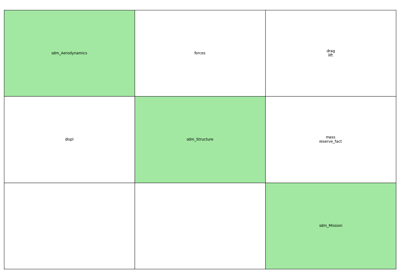gemseo / problems / scalable / data_driven

# problem module¶

## Scalable MDO problem¶

This module implements the concept of scalable problem by means of the ScalableProblem class.

Given

• a MDO scenario based on a set of sampled disciplines with a particular problem dimension,

• a new problem dimension (= number of inputs and outputs),

a scalable problem:

1. makes each discipline scalable based on the new problem dimension,

2. creates the corresponding MDO scenario.

Then, this MDO scenario can be executed and post-processed.

We can repeat this tasks for different sizes of variables and compare the scalability, which is the dependence of the scenario results on the problem dimension.

class gemseo.problems.scalable.data_driven.problem.ScalableProblem(datasets, design_variables, objective_function, eq_constraints=None, ineq_constraints=None, maximize_objective=False, sizes=None, **parameters)[source]

Bases: object

Scalable problem.

Constructor.

Parameters:
• datasets (list(Dataset)) – disciplinary datasets.

• design_variables (list(str)) – list of design variable names

• objective_function (str) – objective function

• eq_constraints (list(str)) – equality constraints. Default: None.

• eq_constraints – inequality constraints. Default: None.

• maximize_objective (bool) –

maximize objective. Default: False.

By default it is set to False.

• sizes (dict) – sizes of input and output variables. If None, use the original sizes. Default: None.

• parameters – optional parameters for the scalable model.

create_scenario(formulation='DisciplinaryOpt', scenario_type='MDO', start_at_equilibrium=False, active_probability=0.1, feasibility_level=0.5, **options)[source]

Create a Scenario from the scalable disciplines.

Parameters:
• formulation (str) –

The MDO formulation to use for the scenario.

By default it is set to “DisciplinaryOpt”.

• scenario_type (str) –

The type of scenario, either MDO or DOE.

By default it is set to “MDO”.

• start_at_equilibrium (bool) –

Whether to start at equilibrium using a preliminary MDA.

By default it is set to False.

• active_probability (float) –

The probability to set the inequality constraints as active at the initial step of the optimization.

By default it is set to 0.1.

• feasibility_level (float) –

The offset of satisfaction for inequality constraints.

By default it is set to 0.5.

• **options – The formulation options.

Returns:

The Scenario from the scalable disciplines.

Return type:

Scenario

exec_time(do_sum=True)[source]

Get total execution time per discipline.

Parameters:

do_sum (bool) –

sum over disciplines (default: True)

By default it is set to True.

Returns:

execution time

Return type:

list(float) or float

plot_1d_interpolations(save=True, show=False, step=0.01, varnames=None, directory='.', png=False)[source]

Plot 1d interpolations.

Parameters:
• save (bool) –

save plot. Default: True.

By default it is set to True.

• show (bool) –

show plot. Default: False.

By default it is set to False.

• step (bool) –

Step to evaluate the 1d interpolation function Default: 0.01.

By default it is set to 0.01.

• varnames (list(str)) – names of the variable to plot; if None, all variables are plotted. Default: None.

• directory (str) –

directory path. Default: ‘.’.

By default it is set to “.”.

• png (bool) –

if True, the file format is PNG. Otherwise, use PDF. Default: False.

By default it is set to False.

plot_coupling_graph()[source]

Plot a coupling graph.

Return type:

None

plot_dependencies(save=True, show=False, directory='.')[source]

Plot dependency matrices.

Parameters:
• save (bool) –

save plot (default: True)

By default it is set to True.

• show (bool) –

show plot (default: False)

By default it is set to False.

• directory (str) –

directory path (default: ‘.’)

By default it is set to “.”.

plot_n2_chart(save=True, show=False)[source]

Plot a N2 chart.

Parameters:
• save (bool) –

save plot. Default: True.

By default it is set to True.

• show (bool) –

show plot. Default: False.

By default it is set to False.

Return type:

None

property is_feasible

Get the feasibility property of the scenario.

property n_calls

Get number of disciplinary calls per discipline.

Returns:

number of disciplinary calls per discipline

Return type:

list(int) or int

property n_calls_linearize

Get number of disciplinary calls per discipline.

Returns:

number of disciplinary calls per discipline

Return type:

list(int) or int

property n_calls_linearize_top_level

Get number of top level disciplinary calls per discipline.

Returns:

number of top level disciplinary calls per discipline

Return type:

list(int) or int

property n_calls_top_level

Get number of top level disciplinary calls per discipline.

Returns:

number of top level disciplinary calls per discipline

Return type:

list(int) or int

property status

Get the status of the scenario.

## Examples using ScalableProblem¶Scalable problem

Scalable problem